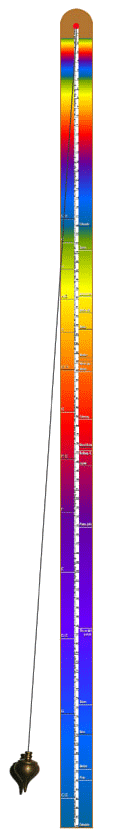### Pendulum for celestial rhythms

For a given length from the suspension to the center of gravity, a pendulum swings back and forth at a given frequency (frequency per unit time).Regardless of whether the pendulum swing is wide or small, the frequency of the back-and-forth swing is always the same for a given length.

The longer a pendulum is, the lower the frequency with which it swings back and forth.

The formula for calculating the so-called mathematical pendulum (string pendulum) was discovered by the Italian scientist Galileo Galilei after he observed the movements of the lamps hung there in different lengths in a church.

He recognized the connection of gravitational acceleration (which is 981 centimeters per second squared on the earth's surface), pendulum length and oscillation time.

A quarter of a pendulum length oscillates with twice the frequency !

##### The pendulum formula

(from the book "Die Kosmische Oktave" by Hans Cousto):

It is

g = 981 cm . sec -2 = Acceleration due to gravity
L = the length of the pendulum (suspension to the center of mass)
π = 3.14159 Pi
T = Duration of a complete oscillation period (back and forth).

It goes:

T = 2 . π . √ (l:g)  =  2 . π . L -0,5 . g0,5

In our case, T and g are known, and the length L is the quantity we are looking for, so the equation must be solved for L. To do this, the equation is first squared:

T2 = 4 . π2 . L . g-1

This equation is now solved for L:

L = T2 . g . π-2 . 0.25  =  T2 . g : (4 . π2)

Any value for T can now be inserted into this equation, whereby the equation can still be transformed expediently beforehand:

L= T2 . g . π-2 . 0.25  =  T2 . 24.85 cm . sec-2

Only the time has to be squared and multiplied by 24.85 cm . sec-2 and the result, the pendulum length, is known. However, one can also divide the number 24.85 cm . sec-2 by the square of the frequency f and likewise obtain the desired pendulum length.

The 16th octave of the synodic solar day corresponds to the time:

T = 86400 sec . 2-16 = 1.31836 sec

and the frequency

f = 86400-1 . 216 = 0.7585 Hz

and so the corresponding pendulum length is:

L = 1.318362 sec2 . 24.85 cm . sec-2 = 43.2 cm

L = 24.85 cm . sec-2 . 0.7585-2sec2 = 43.2 cm

In the table "Planets - Sounds - Colors — Meters" the pendulum lengths of the different cycles of the earth, the moon, the planets and the sun are listed.

With a quarter of the pendulum length a pendulum swings back and forth with double frequency, with four times the length with half frequency !

##### Cosmic Pendulum Mass Tape

to print, cut out and glue (on a cardboard or wooden strip):